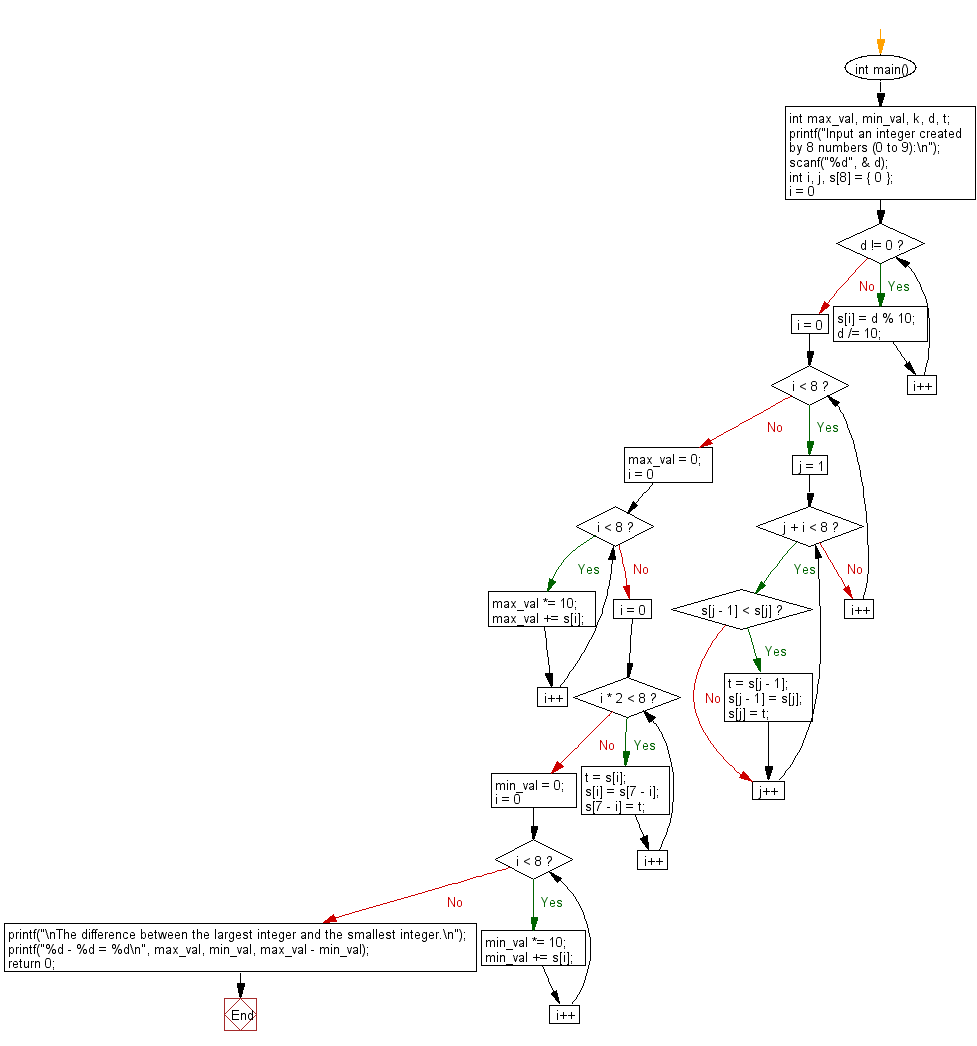﻿ C : Largest, smallest integer created by 8 numbers (0 to 9)

# C Exercises: Find the difference between the largest integer and the smallest integer created by 8 numbers from 0 to 9

## C Basic Declarations and Expressions: Exercise-143 with Solution

Write a C program to find the difference between the largest integer and the smallest integer, which are created by 8 numbers from 0 to 9. The number that can be rearranged shall start with 0 as in 00135668.

Input:
Data is a sequence of 8 numbers (digits from 0 to 9).
Output:
The difference between the largest integer and the smallest integer.

Sample Solution:

C Code:

``````#include<stdio.h>

int main() {
int max_val, min_val, k, d, t;

// Prompt user to input an integer created by 8 numbers (0 to 9)
printf("Input an integer created by 8 numbers (0 to 9):\n");
scanf("%d", &d);

int i, j, s = {0};

// Extract individual digits and store them in array s
for (i = 0; d != 0; i++) {
s[i] = d % 10;
d /= 10;
}

// Sort the digits in descending order
for (i = 0; i < 8; i++) {
for (j = 1; j + i < 8; j++) {
if (s[j - 1] < s[j]) {
t = s[j - 1];
s[j - 1] = s[j];
s[j] = t;
}
}
}

max_val = 0;

// Construct the largest integer from sorted digits
for (i = 0; i < 8; i++) {
max_val *= 10;
max_val += s[i];
}

// Reverse the order of digits in array s
for (i = 0; i * 2 < 8; i++) {
t = s[i];
s[i] = s[7 - i];
s[7 - i] = t;
}

min_val = 0;

// Construct the smallest integer from reversed digits
for (i = 0; i < 8; i++) {
min_val *= 10;
min_val += s[i];
}

// Calculate and display the difference between the largest and smallest integers
printf("\nThe difference between the largest integer and the smallest integer.\n");
printf("%d - %d = %d\n", max_val, min_val, max_val - min_val);

return 0;
}
``````

Sample Output:

```Input an integer created by 8 numbers (0 to 9):
25346879

The difference between the largest integer and the smallest integer.
98765432 - 23456789 = 75308643
```

Flowchart:C programming Code Editor:

What is the difficulty level of this exercise?

Test your Programming skills with w3resource's quiz.

﻿